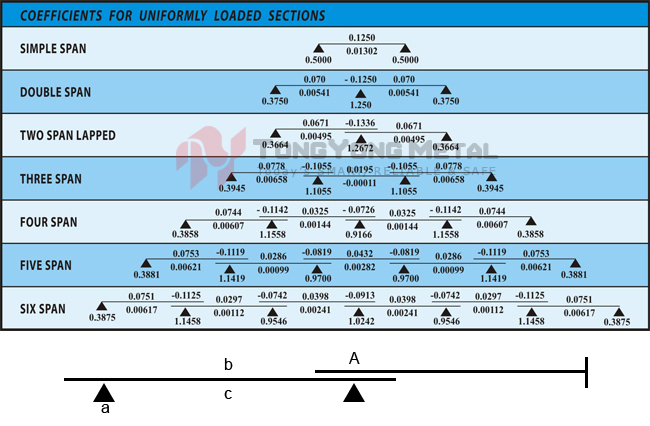# Bending Moment CoefficientsA = Lapped Length = 10% Of Span a = Reaction Coefficient L = Span(mm) R = Reaction = awL N b = Bending Moment Coefficient E = Modulus Of Elasticity = 2x105 N/mm2 M = Moment = bwL2 Nmm c = Deflection Coefficient I = Moment Of Inertia Of Section (mm4) D = Deflection = cwL4/El mm w = Unifromly Distributed Load(N/mm)

Go Back